# A gentle journey from linear regression to neural networks

Source: Deep Learning on Medium

## Soft introduction to some Machine Learning and Deep Learning concepts.

### Introduction

Deep-learning is a very trendy term. The main reason is that related techniques have recently shown incredible ability to produce really good, if not state of the art, results on various kind of problems, ranging from image recognition to text translation. Standing in front of this growing technology, made possible by the increase of data and computer power available, it can be sometimes difficult for non-initiated people to really know “what happens behind the scene”. What is deep-learning? How do neural networks work?

We would like, with this article, to help dissipate most of the confusion or interrogation that can be related to the subject and make place for a correct (even if really basic) understanding and some insightful intuitions.

For this, we will try to give some simple keys to understand what deep learning is and more especially how neural networks work. We will make use of examples, illustrations, analogies and whatever can make the understanding as easy as possible. Our goal here is more to make the main guideline as clear as possible than to obstruct the reader comprehension with some technical details (that can be, nevertheless, very interesting). Especially, we will go from simple problems to more difficult ones in order to show, along the way, how neural networks naturally (at least in the spirit) extend some well known techniques. Obviously, on our way, we will have to make some simplifications or to omit some details. We apologise in advance if it can be, at some points, frustrating for the more advanced readers.

As the purpose of this article is mainly to give to the reader a simple overview and some intuitions to feel confortable with the main ideas of machine learning, deep learning and neural networks, no advanced prior mathematical background is required for most of the parts thereafter.

#### Outline

In the first section, we give a definition of machine learning and show with the very basic example of linear regression how machine learning techniques are aimed at extracting knowledge from data.

Then, in the second section, we show that problems often get harder than the really simple linear regression and that some more advanced machine learning techniques are needed. We introduce the fact that more advanced models, with better predictive power, often come at the price of some higher difficulties in optimisation or interpretability of the model and then discuss briefly these two questions.

Finally, in the last section, we see that for some really complex problems we can choose to drop (partially or fully) interpretability to design models with a better predictive power. We introduce deep learning and more especially neural networks. We show in what sense neural networks can be seen as natural extension of our initial linear regression example and discuss the backpropagation algorithm.

### Basic Machine Learning

#### What is machine learning?

Arthur Samuel, pioneer in the field of computer gaming and artificial intelligence, defined Machine Learning (ML) as the field of study that gives computers the ability to learn without being explicitly programmed. In other words, whatever the purpose of our algorithm is, the rules to achieve this goal are not explicitly programmed but are “learned” (and we will come back to this word later) by the computer, based on some useful data. This is the big difference with a classical algorithm.

In a classical algorithm, rules are explicitly given to the computer to perform a task. In a machine learning algorithm, a model (parametrized or not) that define a family of possible rules is given to the computer along with a whole bunch of data and a strategy to find the better rules among the possible ones based on these data (optimisation). In the next paragraph we will explicit all these elements in one of the most basic machine learning models that exist: the linear regression.

#### The simple linear regression

Linear regression is one of the most simple examples of machine learning algorithms we can think of. Let us see that it perfectly fit the description we gave above. Suppose that you have a bunch of data about houses on two attributes : size (denoted by s) and price (denoted by p). Now, suppose that you want a program that takes as an argument the size of a house and returns a price which is the estimated price of such house. The first option is to program explicitly the rule. In this case it means that we have to define explicitly the function f such that p = f(s). In other words we have to give explicitly the price as a well defined function of the size. However we could have no idea of what this function can be or maybe we could have just a vague idea of it. If so, we can rely on data to build our rule in a ML way. Then, we first define a set (a family) of rules : in this example we suppose that a linear rule expresses the link between price and size. So, we now have that f has the form f(s)=as+b, with a and b unspecified parameters (degrees of freedom) that need to be defined based on the available data and following a given strategy. Classically, for linear regression this strategy is very simple and consists to chose a and b such as to minimize the sum of squared errors between true outputs and predicted outputs. This can be done analytically in the linear regression case (we can find a closed form solution). But we will see that things are not always as easy. However we can notice that we have in this example our three mentioned parts : a (parametrised) model, some data and an optimisation strategy (a way to find the optimal parameters). We will meet again this triptych (model/data/optimisation) in the more advanced methods discussed along this article.

#### Non-parametrised models

The previous example of linear regression is an example of parametrised model, where a and b are the parameters. Along this document we will mainly deal with this kind of models because we want to show the transition up to neural networks which are (highly) parametrised. However one should keeps in mind that there also exist non-parametrised models. Nevertheless, the triptych stays exactly the same. The model still defines a set of possible functions and the selection among this set is done based on available data following a given optimisation strategy (most of the time the minimisation of an error).

### Advanced Machine Learning

#### Problems often get harder

Of course problems can’t always be tackled with a method as simple as linear regression and, in most of the situations, we will have to build more complex models. Some problems do not even fit the specific framework suggested by linear regression (that is : take some real input and return a real output). Nevertheless, whatever the method we choose, we can always recover our underlying triptych model/data/optimisation.

For example, consider that you have some data about the uses that your customers are doing of one of your services and you want a tool to predict if a client is going to churn or not based on these data. In this example the output to predict is not a real value but a binary value (two classes problem) and, so, we face a classification problem instead of a regression problem. Then, the output prediction should be a churn probability between 0 and 1 and the linear regression that has unbounded output should be, for example, turned into a logistic regression by applying a dedicated non-linear function on top of the linear output.

Another example is to consider a customer segmentation problem. Suppose that you have some data about customers and you want to exhibit clusters in these data in order to obtain a customer segmentation. Here the problem is no more a supervised problem. Indeed, we do not want to learn a mapping between some inputs and a given output (supervised) but we look for some structures among unlabelled data (unsupervised).

Even if we are not going to give details about each and every kind of machine learning algorithms, we can give the following big picture : more advanced models are designed to express more complex structures in the data but it can be at the price of some difficulties to optimise or to interpret the model. Let us discuss these two points thereafter.

#### Optimisation

Once the model defined, it has to be optimised to fit the data “just enough” to capture the relevant general structures in the data while letting aside irrelevant specific “noise” (we will discuss later the notion of “overfitting”). Indeed, as it was previously mentioned, a model define a space (of possible “instance” of this model) and we need to find the optimal point in this space with respect to a chosen metric (a way to evaluate the quality of each point in that space). This metric is most often defined by an error term, that penalise instance of the model that doesn’t fit the data enough, and sometimes joined by a regularisation term, that penalise instance of the model that are too complex and too close to the data. Then, we can mainly classify the optimisation problem that we get in three categories.

First of all, for very simple methods, we can face a quadratic-like optimisation problem. Here we mean cases where it is possible to find a closed-form solution to our problem (we know how to mathematically express the solution of the problem). Linear regression is clearly such a method. The fact that we can obtain a closed-form solution is very appealing but also reflect the simplicity, and so the inability to catch complex structures in the data, of the space defined by the model.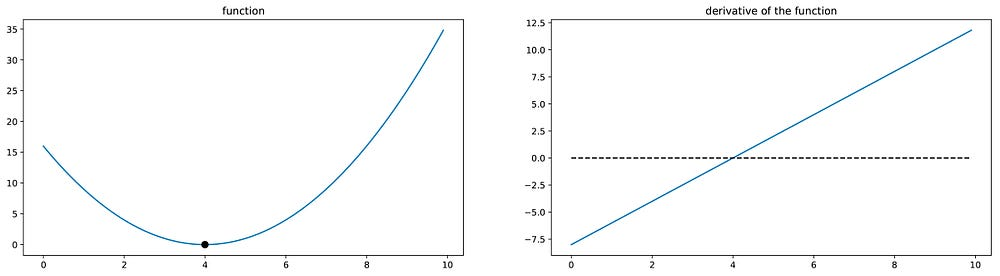Quadratic minimisation with linear derivative. Finding the minimum is straightforward in an analytical way : we just need to find the 0 of a linear function.

Then, the optimisation problem can be non-quadratic but convex. Non-quadratic optimisation problems often can’t be solved in an analytical way and require most of the time an iterative approach. The main idea behind these iterative approaches is to start at a given point of the space — the space described by our model, in which a point is an instance of the model, for example with specific parameters — and to try to improve this solution iteration by iteration choosing, at each iteration, to make a little step in the best possible direction in our space (depending on how we define the notion of “best”). These iterative approaches can take different shapes such as various kinds of gradient descents variants, EM algorithms and others, but at the end the underlying idea is the same : we can’t find direct solution so we start from a given point and progress step by step taking at each iteration a little step in a direction that improve our current solution. See the next figure for an illustration of gradient descent. For such iterative methods, the convexity of the space is a very important property that ensure we will reach the global optimum no matter the chosen starting point (the instance of the model we will obtain will be the best possible with respect to the defined optimisation problem).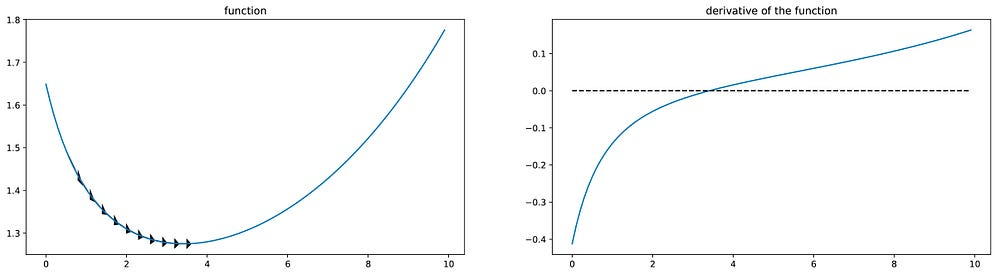Convex minimisation. Finding the minimum is not straightforward and require iterative approach (as the derivative is no longer linear). Here, we used gradient descent. However, convexity ensure that iterative approach will reach the global minimum.

Finally, the space defined by the model can be non-convex and we then face a non-convex optimisation problem. In this case, the non-linearity will again impose to make use of an iterative approach. However the non-convexity makes that we are not sure anymore that the iterative procedure will reach the best possible point over the entire space. In other words the optimum (the instance of the model) we obtain highly depends on the starting point we choose. This is obviously the worst case of optimisation problem we can face and an obvious drawback of the high ability of some more advanced models to express complex structures inside the data.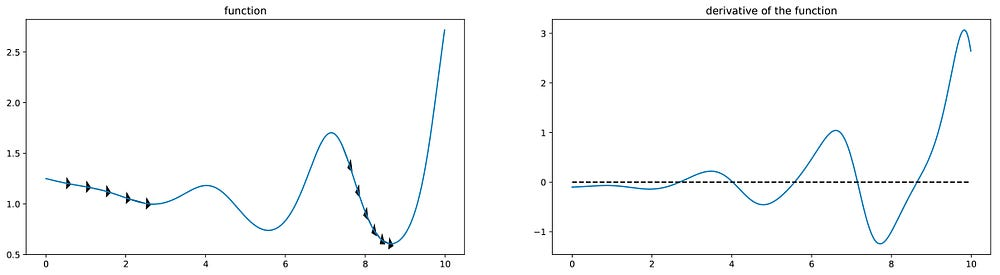Non-convex minimisation. Finding the minimum require iterative approach (here, gradient descent) but the non-convexity make it possible to reach local minima instead of the global minimum depending on the starting point.

#### Be careful about overfitting

In machine learning, in general, when dealing with the optimisation process, we need to be extremely careful about overfitting. We say that the model overfits the data when the model has learned not only the interesting general features that we desire but also some specific undesired noise. In other words, overfitting is when the optimisation process lead to an instance of model that is too close to the training data and, so, that won’t generalise very well to new unseen data. The following figure illustrates pretty well the phenomenon of overfitting.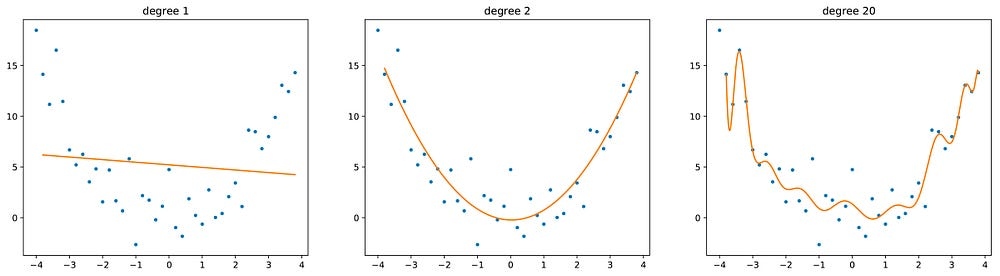From left to right : underfitting, fitting and overfitting. Over the same set of data we fit polynomial functions of various degrees. We can see that 1 degree polynomial function has not enough freedom while 20 degrees polynomial function has too much.

The ratio between the number of parameters of the model and the number of data available for the training has an impact on the overfitting risk. If there are not enough data compared to the number of parameters, there is, in some sense, space for learning undesired noise. But if the number of data is great enough, it will have a regularisation effect and will force the parameters to learn general features only (see the following figure). When fitting a neural network, the number of parameters can be very high and so is the risk of overfitting.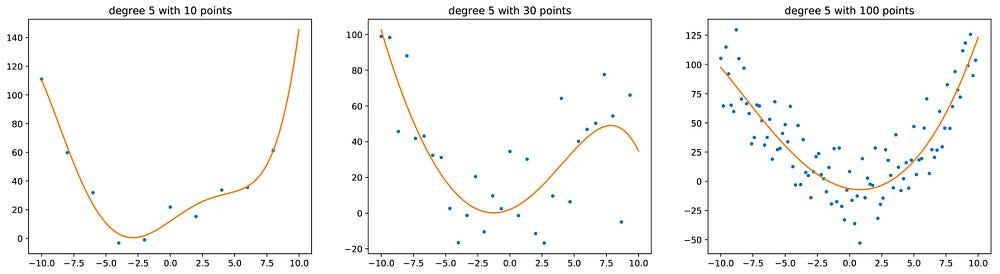From left to right : fitting of a 5 degrees polynomial function with 10, 30 and 100 points. We can see that more points have a regularisation effect : with 100 points, the fitted curve looks like a 2 degrees polynomial function.

We should notice that another way to regularise the parameters/to constraint the parameters to focus on the general features is to introduce explicit regularisers. We won’t give any details about this in this article but it is a very important notion in machine learning in general: the error function (that we desire to optimise) often contains regularisers to enforce the focus on the main desired feature and reduce the potential effect of noise.

#### Model interpretability

The interpretability of a model is a big question that can’t be neglected and is sometimes a determining factor when deciding to choose a model rather than another.

By “interpretability” of the model we mean the ability, once the model optimised, to understand why some inputs give some outputs. Let us take the example of the linear regression of the previous section (p=f(s)=as+b, with s the size of a house and p its price). Once we have optimised parameters a and b, based on the data, we can fully interpret what we obtained. Indeed we can say, in order to guess the price of a new house, that we have a base price of b units of money to which we add a units of money for each unit of size of the house. Here again, the full interpretability of linear regression comes from its over-simplicity and along with some limitations in terms of modeling. At the opposite, some models are very powerful but far less (if any) interpretable. However, there is not necessarily a direct link between performance of a model and its interpretability. SVMs are, for example, known to perform well on many problems and their interpretability can be also pretty good.

#### Importance of interpretability

Notice that, with the more and more important place that Machine Learning is going to take in our daily lives, the question of interpretability is becoming central and will be more and more in the future. Machine Learning models are going to assist humans for some (possibly important) tasks (in health, finance, driving…) and we sometimes want to be able to understand how the results returned by the models are obtained. For example, a smart keyboard that suggest next most probable words when typing a message doesn’t necessarily need to be understandable : we just want it to be efficient. However, a model that predict the presence of a disease or not for a patient better be interpretable on top of accurate : in this case we are not only interested by the result but we want to understand the “logic” behind it in order to let a human confirm, or not, the diagnosis.

So, interpretability is a very appealing feature for a model. However, sometimes it’s necessary to trade some (if not all) interpretability for a greater predictive power by setting up some very complex models : that is exactly what is done in the case of neural networks discussed in the following section.

### Deep Learning

#### What are we talking about?

A quick search on Google give us the following definition of “deep learning” : “the ensemble of deep learning methods is a part of a broader family of machine learning methods that aims at modelling data with a high level of abstraction”. Here, we should understand that deep learning consists in setting up very complex models, trading-off ease of interpretability for predictive power.

Neural networks belong to deep learning methods. In the very big picture, they are highly parametrised complex functions that we try to optimise (we search for the best parameters) to fit our data. If we want to schematise at extreme, we could say that neural networks are the very complex “evolution” of linear regression designed to be able to model complex structures in the data.

Let us consider, for example, a regression or a classification problem. In both cases we have some inputs, denoted by (i1, i2, …, in), and we want to find a function of these inputs that explain pretty well the observed corresponding outputs, denoted by (o1, o2, …, om). In other words, as in the case of our linear regression example, we are looking for a function f such that (o1, o2, …, om) is well approximated by f(i1, i2, …, in). The idea behind neural networks modelling is to forget the idea to set up a lightly parametrised function mainly “shaped” by human and adjusted by the machine (through these few parameters, as in our linear regression example) but instead to set up a highly parametrised function very flexible that doesn’t make too much sense a priori for human but that will be shaped conveniently during the learning phase. Let us try to illustrate this on a simple neural network.

#### The basic Forward Neural Network

In this subsection, we will take a look at the basic forward neural network. It will give us the opportunity to introduce some basic terminology about neural networks and to see clearly how they can be seen as a natural extension of the linear regression.

Then, let us consider again our house pricing example, but this time with 2 inputs available (let’s denote these inputs i1 and i2), and no special knowledge about the relationship between these inputs and the price that we want to predict (denoted by o1) except that this relationship is, a priori, pretty complex and non-linear.

So we want to learn the function f such that f(i1, i2) is a good estimator of o1. We could then suggest the following first model:

where w11 and w12 are just weights/coefficients (do not take care about the indices for now). Before going any further, we should notice that, here, there is no constant term in the model. However, we could have introduced such term by setting f1(i1, i2) = w11 * i1 + w12 * i2 + c. In order to shorten a little some notations, we won’t write such constant terms in the following but the reader should keep in mind that they can always be added (and most often are).
Such model is a linear regression model for multiple inputs (also called multilinear regression) and can be represented as follows.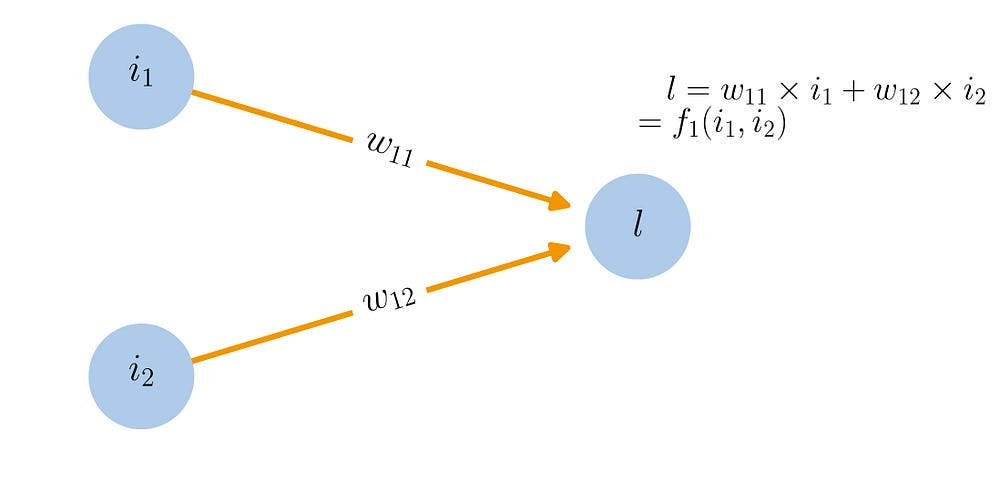“Network” graphical representation of the multilinear regression (model f1)

In this case, the model is easy to understand and to fit but has a big drawback : there is no non-linearity ! This obviously do not respect our non-linear assumption. In order to introduce a non-linearity, let us make a little modification in the previous model and suggest the following one.

where a(.) is a function called “activation function” which is non-linear. Then, we can notice that w11 * i1 + w12 * i2 is still linear, but as we make this value goes through a non-linear function, the overall result is no longer linear and, so, this model is closer to our assumption than the previous model. This model can be represented as follows.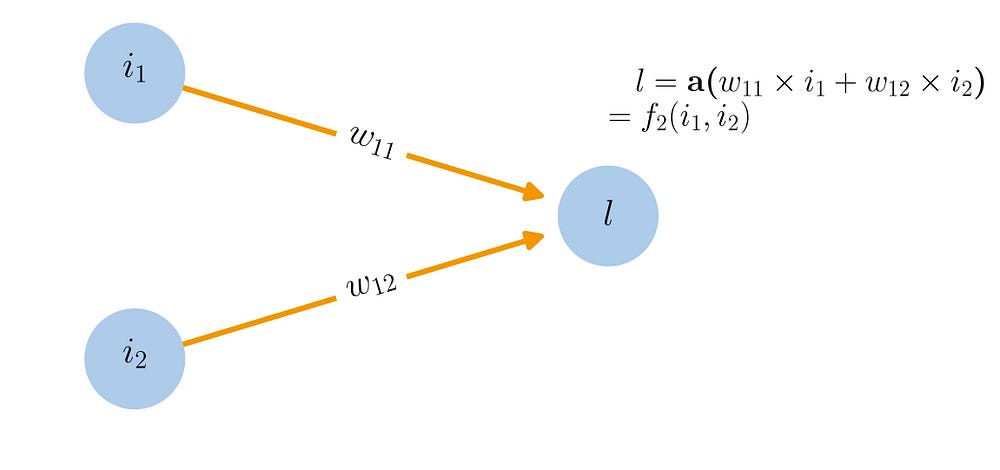“Network” graphical representation of the f2 model. If the activation function a is the logistic function, it defines the well known logistic regression model.

Notice that if we choose as activation function the so called “logistic function”, we define this way a model called “logistic regression” that can fit, for example, some binary classification problems (indeed the logistic function outputs a number between 0 and 1 that can then be seen as a probability to be in one of the two classes).

However, even if better than multilinear model, this model is still too simple and can’t handle the assumed underlying complexity of the relationship between inputs and output. We can make a step further and enrich the model the following way. First we could consider that the quantity a( w11 * i1 + w12 * i2 ) is no longer the final output but instead a new intermediate feature of our function, called l1. Second we could consider that we build several (3 in our example) such features in the same way, but possibly with different weights and different activation functions : l1 = a11( w11 * i1 + w12 * i2 ), l2 = a12( w21 * i1 + w22 * i2 ) and l3 = a13( w31 * i1 + w32 * i2 ), where the a’s are just activation functions and the w’s are weights. Finally, we can consider that our final output is build based on these intermediate features with the same “template” : a2( v1 * l1 + v2 * l2 + v3 * l3 ). If we aggregate all the pieces, we then get :

where we should mainly keep in mind that a’s are non-linear activation functions and w’s and v’s are weights. In the next figure, we give the network graphical representation of this model in the same way as we did for the previous ones.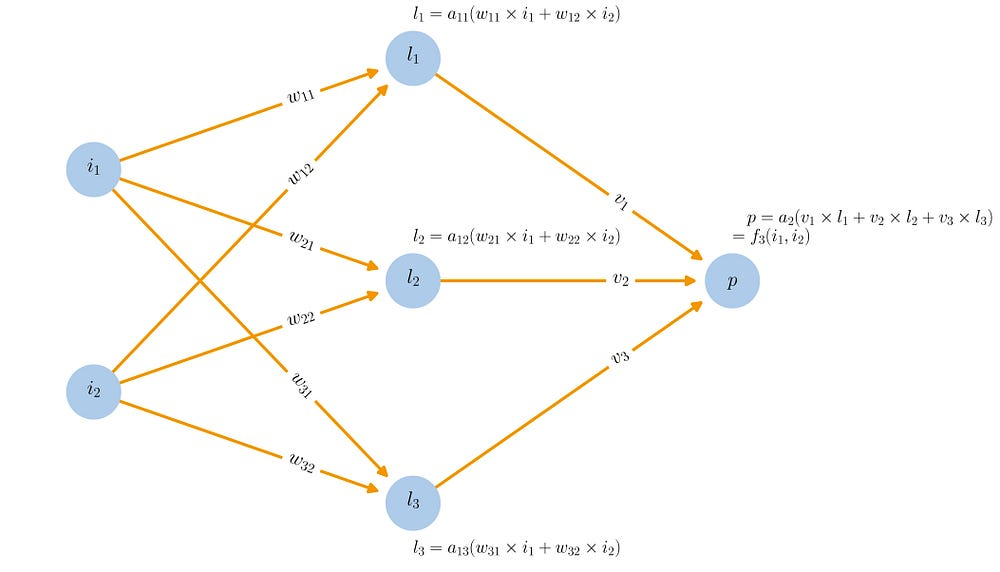“Network” graphical representation of the f3 model. Here we have a neural network with 2 entries, 1 hidden layer with 3 hidden nodes/neurones and 1 output.

This last model is a basic feedforward neural network with 2 entries (i1 and i2), 1 hidden layer with 3 hidden neurones (whose outputs are l1, l2 and l3) and 1 final output. We could have decided to add another intermediate “hidden” layer between the l’s and the final output exactly in the same way as we just add these l’s between the input and the output : then we would have had a neural network with 2 hidden layers. Or we could have chosen to stay with 1 hidden layer but to have more neurones in it (5 instead of 3, for example). So, we have different ways to enrich/complexify the model that will make the number of weights grow. The number of hidden layers, the number of neurones per layers such as the values of weights and the nature of activation functions define the neural network and, so, the “template” of the function described by this network.

Finally, let us notice a few important things. First, on the way to mathematically describe these networks, we can (and we always do !) write all the previous equations with matrix notations : it makes all this a lot cleaner to read for big architectures. However, here, our example is small enough to afford not to use matrix notations and, so, we can avoid them for readers potentially not at ease with these notations. Second, all the neural networks do not fit the template described above : there are different kinds of architectures (we will say a few words about it in the next paragraph), but the feedforward neural network is really the first basic architecture to understand. Third, once defined, the model still need to be fitted (the weights should be adjusted, based on the data, to minimise some error function, just as in the case of the linear regression) and that is a really difficult optimisation task to complete.

#### Different needs, different architectures

As we have seen in the previous paragraph, neural network are templates of functions highly adjustable, through their parameters, that need to be optimised to fit the data. But depending on the nature of the problem and on the data to model, we maybe want to use different kind templates. These different kind of templates are called different “architectures”. For example, the basic Forward Neural Network (also called Multi Layer Perceptron) discussed above is the first basic architecture. However there exist several others. Among the well known architectures, we can mention Recurrent Neural Networks (RNN) — that represent a recurrent function of some sequential data where an output at time t depends on the input at this time t and on the previous output at time t-1 — and Convolutional Neural Networks (CNN) — that represent the mathematical convolution operation on the inputs and show good properties for example in some image based problems such as image recognition.
With all the ongoing research works, there are always more architectures that are imagined depending on the problem to model. Obviously, we can’t describe all these kinds of architectures (and it would be completely out of the scope of this article) but the most important thing to keep in mind here is that an architecture of neural network should always be seen as a space of possible functions where optimising the parameters of the network is equivalent to find the best function in this space (based on an optimality criterion). So, it is of course important to chose the right architecture because if not well chosen we will define a space in which even the best function could be far from what we expect as a result.

#### Why is it a good idea?

Among the reasons that make neural networks so effective and popular, we can mention the following three. First, the always increasing volume of data available in many areas makes reasonable the use of such highly parametrised models. Notice that the parameters of the model are also called “degrees of freedom” (this term is not specific to machine learning field and express the notion of “adjustable values” or “action levers” in a model) and that a lot of degrees of freedom in a model require a lot of data to adjust/calibrate this model. In the same spirit as you can’t afford in a linear system of equations to have more unknowns than equations, you need more data than parameters in your network (in fact, a lot more is better). Second, the compute power, which is always greater, coupled with smart optimisation techniques makes possible to train at very large scale models with so much parameters. In large models, there can be millions of parameters to optimise and a minimum of compute power is required to achieve the optimisation process in a reasonable time. Finally, as we just said, the optimisation process is a difficult task and, with no doubt, simple but smart techniques of optimisation, such as the well known “backpropagation” that suits perfectly the framework of a computer based optimisation, are one of the main explanations of the success met by neural networks.

#### Neural networks are hard to optimise

So, what is backpropagation? Before answering this question, let us discuss briefly the kind of optimisation problem that we need to face when dealing with neural networks. We are looking for the best function (for the parameters that minimise some predefined error metric) among the space of functions described by the chosen “architecture”. Due to the non-linear activation functions that compose this “architecture” and due to the overall complexity of the parametrised function defined by the model, the minimisation problem that we face is non-linear and non-convex.

The non-linearity of the optimisation problem requires the use of some iterative approach as described previously. So the idea is the following : set the initial weights of the network randomly (given some well chosen distribution) and then apply a gradient descent method over all these parameters to iteratively improve (reduce) the error metric. Stated in another way it means : make a forward pass through the network with your data, observe the result, compare the model output result with the real expected result, then change a little bit the weights in the network in a way that make the result slightly closer to the true value (through a gradient descent approach) and repeat this process as long as we can make “little moves” of our weights that improve the result.

Let us take an example to make it clearer. Consider the simple forward neural networks presented previously (with 2 entries, 1 hidden layer with 3 hidden neurones and 1 output). Suppose that weights of this neural networks are in some state at a given time (see the next figure). Then, we can compute, for each input in the dataset the predicted output with current weights.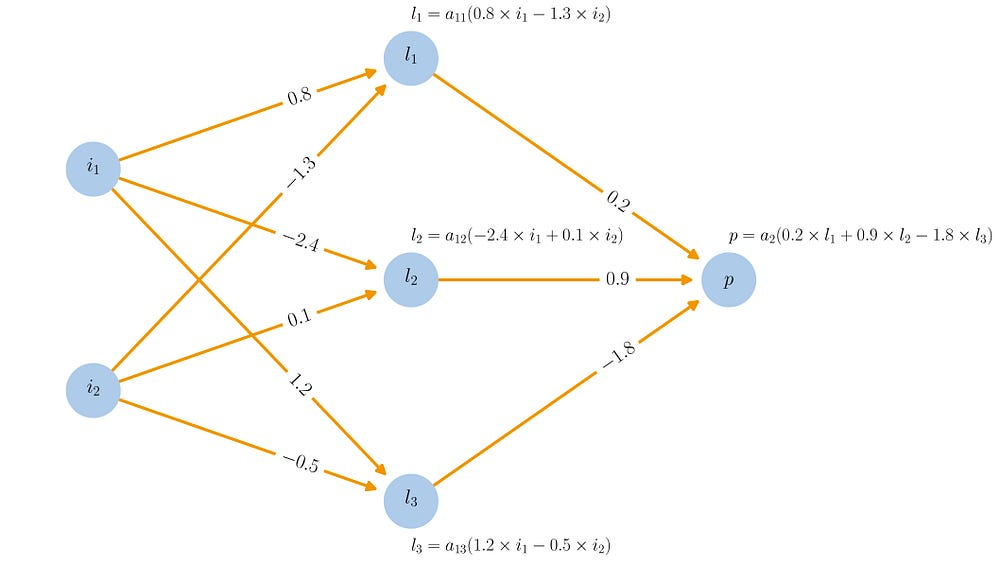At the beginning of a step, the neural network has its weights set at some values. We can then compute a forward path for all inputs (i1, i2).

Now, in order to make one step of gradient descent, we should ask ourselves for each weight in the network independently : what action is going to lower the error between increasing and decreasing a little bit this weight.

Let us emphasise the notion of “independently”: it means that, for each weight, we have to define, assuming all the other weights fixed, whether we need to lower or to increase this weight in order to reduce the error.

Doing so, we attribute in some sense a little “+𝜶_w” (increase) or “𝜶_w” (decrease) to each weight w, where 𝜶_w is positive and is related to how much increasing (or decreasing) this weight will reduce the error (see next figure). The previous quantities are obviously the derivatives with respect to each weights, up to a sign (derivatives would indicate how to increase the error).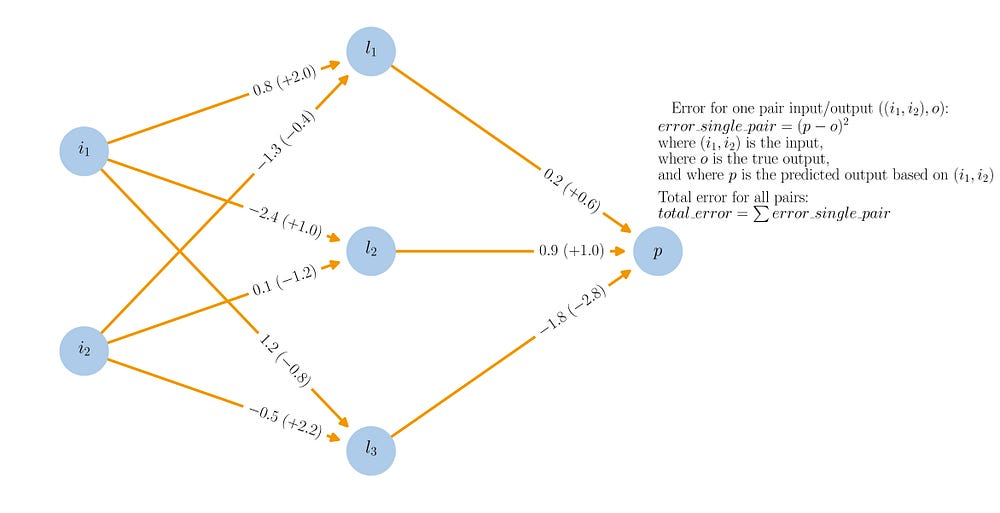Once the forward computations achieved, the error related to each input can be computed. The total error is the sum of the individual errors for each input data. For each weight, we can assign (between parenthesis on the graphic) the opposite sign of the derivative of the total error with respect to this weight. Roughly speaking, for each weight, these values answer the question : if we suppose all the other weights fixed, should we increase or decrease this weight to lower the total error ?

Once it has been done for every weights, we can apply the gradient descent step and all the weights labelled with a “+𝜶_w” are updated such that w w +𝜶_w * step_size whereas all the weights labelled with a “-𝜶_w” are updated such that w w -𝜶_w * step_size (see next figure). Notice that step_size defines how much weights are changed at each iteration and can evolve over the training time. Once the step achieved, we can restart a new iteration, and this until weights can not be improved anymore.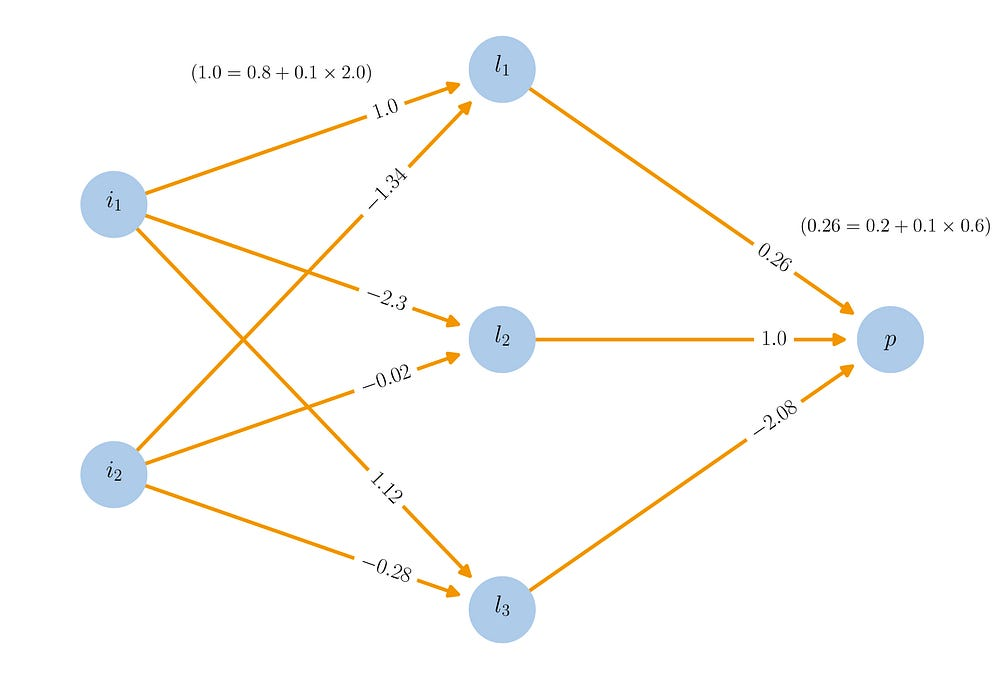Finally, we can apply the gradient descent step. We considered in this example a step size of 0.1. The weights are then updated (two examples are detailed in the graphic) and we can then start again, until weights can not be improved anymore.

Even if we won’t give any more details about it, we should mention that, as for most of the iterative optimisation processes, nothing can ensure that we end an iteration with a better state of the network (with respect to the error) than the initial state (especially because of the step size that can be too large or because we compute update values for each weight supposing all the other weights fixed but we update all the weights at the same time).

#### Backpropagation as a “layer by layer” gradient descent

We just described the gradient descent process over a neural network. However, in order to be able to make these “little moves” of the weights, we still need to compute the derivatives of the error term with respect to each of the weights in the network (we need to know for each weight if we should increase it or decrease it a little). It requires to compute the derivative of the function represented by the network with respect to each of the weights. This is a real big task except if we take advantage of the layered structure of the network to help us. Indeed, as we mentioned earlier, network are often build with layers and it means that we are facing (highly) compositional functions. Back propagation algorithm consists in using this specific kind of layered structure to make the computation of derivatives efficient. For this, we rely on the fact that the derivatives with respect to the weights at a given layer can be expressed as a function of two things : first, the derivatives with respect to the weights of the next level and, second, the value that take the neurone during the forward pass. This particular expression of the derivatives in the networks directly comes from the mathematical derivative of a compositional function (f(g(x))’ = f’(g(x))*g’(x)). Then, efficient derivative computation is done as follows. We start by computing the forward pass (we feed the the inputs and run the computations up to the output). Then we go back in the opposite direction and first compute the derivatives of the error with respect to the weights of the last layer. Based on these computations we can compute the derivatives with respect to the the previous layer and so on, going back up to the first layer. In other words, we “backpropagate” the derivatives computations in the network. This algorithmic technique makes the optimisation process much more tractable.

#### Local minima and overfitting risk

Finally, let us say that the quality of solutions obtained through the optimisation process for neural networks is largely impacted by the non-convexity of the underlying optimisation problem and by the overfitting risk due to the high number of parameters in the model.

Due to the high complexity of the optimisation problem, it is almost impossible that iterative approaches could lead to the global optimum. The non-convexity of the optimisation problem faced brings a big risk to stay stuck in a local minimum during the iterative process. For this reason (and also to tackle computational constraints) stochastic gradient descent (SGD) can also be used. SGD consists in not using the whole training dataset to make the weights updates (through the gradient descent) but instead to use successively different batch of this data in order to make the optimisation process a little bit noisy and to have a chance to overcome, thanks to this noise, local optima. We won’t go any further about the notion of stochastic gradient descent here.

Moreover, when fitting/training a neural network, we must be especially careful about the risk of overfitting. In order to avoid overfitting, we can reduce the number of parameters of the networks, get more data or use explicit regularisers (in the loss function or through a dropout process that we won’t discuss in this article).

### Takeaways

In this modest article we tried to give the reader some intuitions about what neural networks are. For this, we went from the notion of Machine Learning and its simple models to the more specific concept of Deep Learning underlying Neural Networks. Along the way, we encountered various concepts related to Machine Learning such as iterative optimisation, overfitting risks and others. As we mentioned in the introduction, the purpose here was not at all to explore deeply each and every part of the problem but instead to give the big picture of “why”, “what” and “how”.

The main takeaways are the followings:

• Machine Learning is the field of study that gives computers the ability to learn without being explicitly programmed.
• Machine Learning relies on the triptych model/data/optimisation.
• When setting up more complex models, there are mainly two points to take care of : how to optimize and how to interprete?
• Coarsely speaking, when using Deep Learning and Neural Networks we abandon interpretability for a greater predictive power by setting up very complex models.
• The success met by Neural Networks mainly comes from the always increasing volume of available data and compute power and from the very effective backpropagation idea that makes the optimisation process much more tractable.

To conclude, let us say that neural networks are very powerful tools. They have demonstrated their abilities to perform very well on a great number of modern problems. However, they are not magic tools able to solve any kind of problems. The real smart part of the job is still in the hand of humans to know where and how to use these models in a correct way. If it would be offensive to reduce neural networks to “curve fitting” because of all the smart ideas that many people introduced in the field to tackle problems more and more complex, it would also be inaccurate to describe them as “magical tools” because of the obvious limited scope of problem covered by these models for now. As a right balance, let’s enjoy the great (huge!) progresses bring by these tools in many fields while keeping in mind their current limitations… and without being scared by dreadful robot-killers. For now.

Thanks for reading!

Thanks to Baptiste Rocca and Benjamin Blanc for reading the draft and suggesting helpful corrections and improvements.

Any feedback about this article is really welcome!